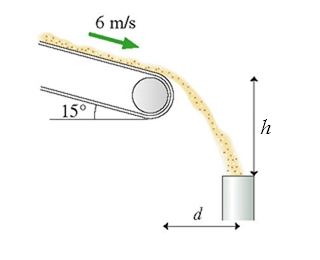Physics Practice Problems Projectile Motion: Horizontal & Negative Launch Practice Problems Solution: Sand moves without slipping at 6.0 m/s down a conv...

🤓 Based on our data, we think this question is relevant for Professor Griffith's class at MC MARICOPA.

# Solution: Sand moves without slipping at 6.0 m/s down a conveyer that is tilted at 15°. The sand enters a pipe h = 3.6 m below the end of the conveyer belt, as shown in the figure: What is the horizontal distance d between the conveyer belt and the pipe?

###### Problem

Sand moves without slipping at 6.0 m/s down a conveyer that is tilted at 15°. The sand enters a pipe h = 3.6 m below the end of the conveyer belt, as shown in the figure:

What is the horizontal distance d between the conveyer belt and the pipe?###### Solution

This problem is asking us to find the horizontal distance traveled by the grains of sand given the magnitude and direction of the initial velocity and the height they fall.

For projectile motion problems in general, we'll follow these steps to solve:

1. Identify the target variable and known variables for each direction—remember that only 3 of the 5 variablesx or Δy, v0, vf, a, and t) are needed for each direction. Also, it always helps to sketch out the problem and label all your known information!
2. Choose a UAM equation—sometimes you'll be able to go directly for the target variable, sometimes another step will be needed in between.
3. Solve the equation for the target (or intermediate) variable, then substitute known values and calculate the answer.

The four UAM (kinematics) equations are:

We define our coordinate system so that the +y-axis is pointing upwards and the +x-direction is horizontal along the launch direction. That means ay = −g, and ax = 0 (because the only acceleration acting on a projectile once it's launched is gravity.)

For projectiles with a negative launch angle, we also need to know how to decompose a velocity vector into its x- and y-components:View Complete Written Solution

Projectile Motion: Horizontal & Negative Launch

Projectile Motion: Horizontal & Negative Launch

#### Q. An electromagnet on the ceiling of an airplane holds a steel ball. When a button is pushed, the magnet releases the ball. The experiment is first done...

Solved • Mon Oct 29 2018 17:47:09 GMT-0400 (EDT)

Projectile Motion: Horizontal & Negative Launch

#### Q. A baseball player friend of yours wants to determine his pitching speed. You have him stand on a ledge and throw the ball horizontally from an elevati...

Solved • Mon Oct 29 2018 17:47:02 GMT-0400 (EDT)

Projectile Motion: Horizontal & Negative Launch

#### Q. You are watching an archery tournament when you start wondering how fast an arrow is shot from the bow. Remembering your physics, you ask one of the a...

Solved • Mon Oct 29 2018 17:46:43 GMT-0400 (EDT)

Projectile Motion: Horizontal & Negative Launch

#### Q. A block of mass m = 2.00 kg is released from rest at h = 0.500 m above the surface of a table, at the top of a θ = 30.0° incline as shown in Figure P5...

Solved • Mon Oct 29 2018 16:36:23 GMT-0400 (EDT)